Prerequisite: Facebook API | Set-1, Set-2

1. search
2. get_connections
3. get_allconnections

## `search `method:

The valid value types are place and placetopic.

Parameters:
id: It is a string containing a valid value.
args: This is optional and they need to passed a query params.

Example: Get details of all places that are near Connaught Place Delhi. The Latitude and Longitude of Connaught Place are – 28.6304, 77.2177

 `import` `json ` `import` `facebook ` ` `  `def` `main(): ` `    ``token ``=` `"Please replace with your access token"` `    ``graph ``=` `facebook.GraphAPI(token) ` ` `  `    ``places ``=` `graph.search(``type` `=``'place'``, center ``=``'28.6304, 77.2177'``,  ` `                                            ``fields ``=``'name, location'``) ` ` `  `    ``for` `place ``in` `places[``'data'``]: ` `        ``print``(``'%s %s'` `%``(place[``'name'``].encode(), place[``'location'``].get(``'zip'``))) ` `     `  `if` `__name__ ``=``=` `'__main__'``: ` `    ``main() `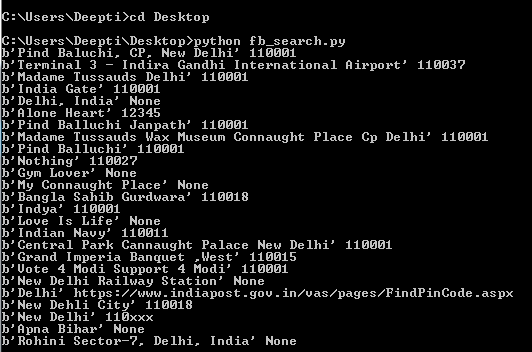Please refer to the link Search Reference for complete list of fields that can used.

## `get_connections `method :

This methods aims at returning all connections or we can say Edges for the mentioned object as dict.

Parameters:
id:A string specifying the unique id for the resource under question.
connection name: A string specifying the connection or edges between the objects.

If connection name parameter is left empty then `get_connections `method will simply return the basic information of the authenticated user.

Example #1: We want to find out the total count of the number of Friends of the active user.

 `import` `json ` `import` `facebook ` ` `  `def` `main(): ` `    ``token ``=` `"Please replace this with your access token"` `    ``graph ``=` `facebook.GraphAPI(token) ` `    ``friends ``=` `graph.get_connections(``id` `=``'me'``, connection_name ``=``'friends'``) ` `    ``print``(json.dumps(friends, indent ``=` `4``)) ` ` `  `if` `__name__ ``=``=` `'__main__'``: ` `    ``main() `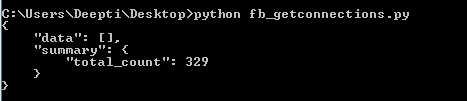Example #2: We want to get list of all posts from a page. For this, we will be using get_connections and later on, we will demonstrate how to use `get_all_connections `method.

 `import` `json ` `import` `facebook ` ` `  `def` `main(): ` `    ``token ``=` `"Please replace this with your PAGE Access Token"` `    ``graph ``=` `facebook.GraphAPI(token) ` `    ``posts_25 ``=` `graph.get_connections(``id` `=``'PAGE_ID'``, connection_name ``=``'posts'``, ` `                                                  ``fields ``=``'id, created_time'``) ` ` `  `    ``print``(json.dumps(posts_25, indent ``=` `4``)) ` ` `  `if` `__name__ ``=``=` `'__main__'``: ` `    ``main()  `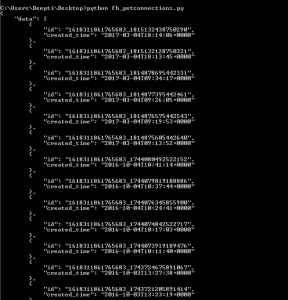Note: This example by default print latest 25 posts. You can add a filter for the post using limit(…) to set the limit for the number of the posts.

Example #3: In this example we will use get_connections method to print all the comments for the posts in `reverse_chronological `order, show sub comments including hidden comments and also show the total count of the comments.

 `import` `json ` `import` `facebook ` ` `  `def` `main(): ` `    ``token ``=` `"Please replace this with your PAGE Access Token"` `    ``graph ``=` `facebook.GraphAPI(token) ` `    ``posts_25 ``=` `graph.get_connections(``id` `=``'POST_ID'``, connection_name ``=``'comments'``,  ` `                                     ``include_hidden ``=` `True``, order ``=``'reverse_chronological'``, ` `                                     ``filter` `=``'stream'``, summary ``=``'total_count'``) ` ` `  `    ``print``(json.dumps(posts_25, indent ``=` `4``)) ` ` `  `if` `__name__ ``=``=` `'__main__'``: ` `    ``main()  `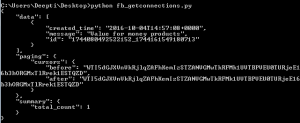## `get_all_connections `method :

Iterates over all pages returned by a get_connections call and yields the individual items.

Parameters:
id:A string specifying the unique id for the resource under question.
connection name: A string specifying the connection or edges between the objects.

Example #1: In this example I have used get_all_connections to list all the posts from a Page listed using the since datetime parameter.

 `import` `json ` `import` `facebook ` `from` `datetime ``import` `datetime ` `  `  `def` `main(): ` `    ``token ``=` `"Please replace this with your PAGE Access Token"` `    ``graph ``=` `facebook.GraphAPI(token) ` `    ``posts_all ``=` `graph.get_all_connections(``id` `=``'PAGE_ID'``, connection_name ``=``'posts'``, ` `                                           ``fields ``=``'created_time, id'``, ` `                                           ``since ``=` `datetime(``2017``, ``1``, ``1``, ``0``, ``0``, ``0``)) ` ` `  `    ``for` `ind, post ``in` `enumerate``(posts_all): ` `     ``print``(json.dumps(ind, indent ``=` `4``)) ` `     ``print``(json.dumps(post, indent ``=` `4``)) ` `     `  `  `  `if` `__name__ ``=``=` `'__main__'``: ` `    ``main() `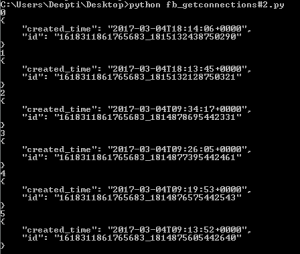References:

My Personal Notes arrow_drop_upCheck out this Author's contributed articles.

If you like GeeksforGeeks and would like to contribute, you can also write an article using contribute.geeksforgeeks.org or mail your article to contribute@geeksforgeeks.org. See your article appearing on the GeeksforGeeks main page and help other Geeks.

Please Improve this article if you find anything incorrect by clicking on the "Improve Article" button below.

Article Tags :

Be the First to upvote.

Please write to us at contribute@geeksforgeeks.org to report any issue with the above content.